# 代码详解：如何用Python快速制作美观、炫酷且有深度的图表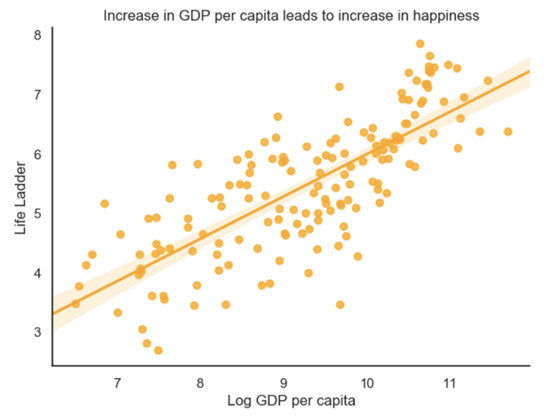《世界幸福报告》试图回答世界范围内影响幸福的因素。图片来源：Nik MacMillan/Unsplash

·     我使用Python进行绘图的经历
·     分布的重要性
·     加载数据和包导入
·     迅速：使用Pandas进行基本绘图
·     美观：使用Seaborn进行高级绘图
·     精彩：用plotly创造精彩的互动情节# 1. 我使用Python进行绘图的经历图片来源：Krys Amon/Unsplash

Matplotlib

Seaborn

Bokeh

Plotly

Kepler.gl (地理空间数据优秀奖)

Kepler.gl不是一个Python库，而是一款强大的基于web的地理空间数据可视化工具。只需要CSV文件，就可以使用Python轻松地创建文件。试试吧!# 2. 分布的重要性图片来源：Jonny Caspari/Unsplash# 3. 加载数据和包导入# Loadthe datadata = pd.read_csv('https://raw.githubusercontent.com/FBosler/AdvancedPlotting/master/combined_set.csv')#this assigns labels per yeardata['Mean Log GDP per capita']  =data.groupby('Year')['Log GDP per capita'].transform(    pd.qcut,    q=5,   labels=(['Lowest','Low','Medium','High','Highest']))

·     年份：计量年（2007 -2018）
·     人均GDP：根据世界银行2018年11月14日发布的《世界发展指标》（WDI），将人均GDP调整为PPP（2011年不变价国际元）
·     社会支持：对下面问题的回答：“遇到困难时，是否可以随时获得亲戚或朋友的帮助？
·     出生时预期健康寿命：出生时预期健康寿命是根据世界卫生组织（WHO）全球卫生观察站（GHO）数据库构建的，数据分别来自2005年、2010年、2015年和2016年。
·     自由选择权：回答下面这个问题：“你是否对自己生活的选择自由感到满意？
·     慷慨：对“过去一个月是否给慈善机构捐过款?”与人均GDP相比
·     政治清廉：回答“腐败现象在政府中是否普遍？”“腐败在企业内部是否普遍？
·     积极影响：包括前一天快乐、欢笑和享受的平均频率。
·     负面影响：包括前一天焦虑、悲伤和愤怒的平均频率。
·     对国家政府的信心：不言自明
·     民主质量：一个国家的民主程度
·     执行质量：一个国家的政策执行情况
·     Gapminder预期寿命：Gapminder的预期寿命
·     Gapminder人口: 国家人口

import plotlyimport pandas as pdimport numpy as npimport seaborn as snsimport plotly.express as pximport matplotlib%matplotlib inlineassertmatplotlib.__version__ == "3.1.0","""Please install matplotlib version 3.1.0 by running:1) !pip uninstall matplotlib2) !pip install matplotlib==3.1.0"""# 4. 迅速：使用Pandas进行基本绘图图片来源：Marvin Meyer/Unsplash

Pandas有内置的绘图功能，可以在Series或DataFrame上调用。之所以喜欢这些绘图函数，是因为它们简洁、使用合理的智能默认值、很快就能给出进展程度。

np.exp(data[data['Year']==2018]['LogGDP per capita']).plot(    kind='hist')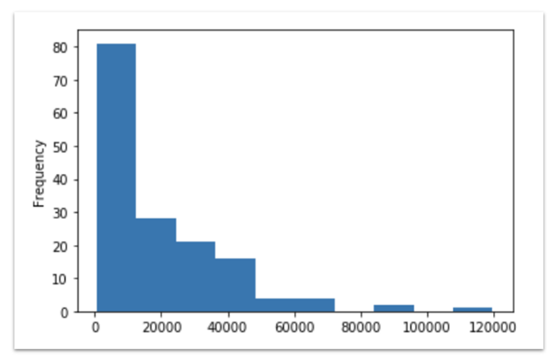2018年：人均GDP直方图。大多数国家都很穷，这一点也不奇怪！

·     kind：Pandas必须知道需要创建什么样的图，可选的有以下几种：直方图（hist），条形图（bar），水平条图（barh），散点图（scatter），面积（area），核密度估计（kde），折线图（line），方框（box），六边形（hexbin），饼状图（pie）。
·     figsize：允许6英寸宽和4英寸高的默认输出尺寸。需要一个元组（例如，我就经常使用figsize=(12,8)）
·     title：为图表添加一个标题。大多数情况下，可以用这个标题来标明图表中所显示的内容，这样回过头来看的时候，就能很快识别出表的内容。title需要一个字符串。
·     bins:直方图的bin宽度。bin需要一个值的列表或类似列表序列（例如， bins=np.arange(2,8,0.25)）
·     xlim/ylim: 轴的最大和最小默认值。xlim和ylim都最好有一个元组（例如， xlim=(0,5)）

data[    data['Year'] == 2018].set_index('Country name')['Life Ladder'].nlargest(15).plot(    kind='bar',    figsize=(12,8))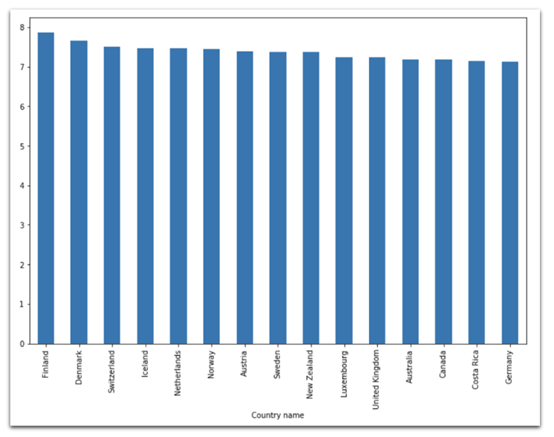2018年：芬兰位居15个最幸福国家之首

np.exp(data[    data['Year'] == 2018].groupby('Continent')['Log GDP per capita']\       .mean()).sort_values().plot(    kind='barh',    figsize=(12,8))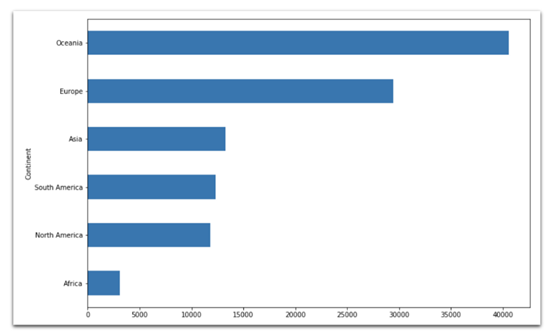data['Life Ladder'].plot(    kind='box',    figsize=(12,8))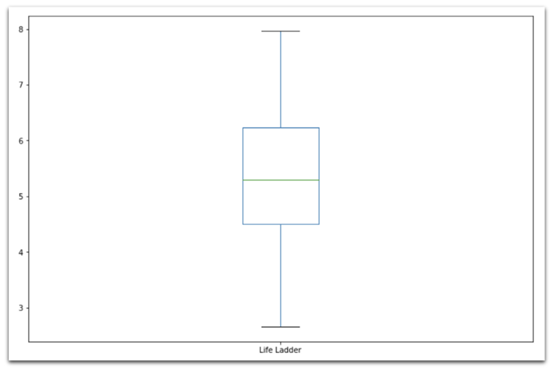data[['Healthy life expectancyat birth','Gapminder Life Expectancy']].plot(    kind='scatter',    x='Healthy life expectancy at birth',    y='Gapminder Life Expectancy',    figsize=(12,8))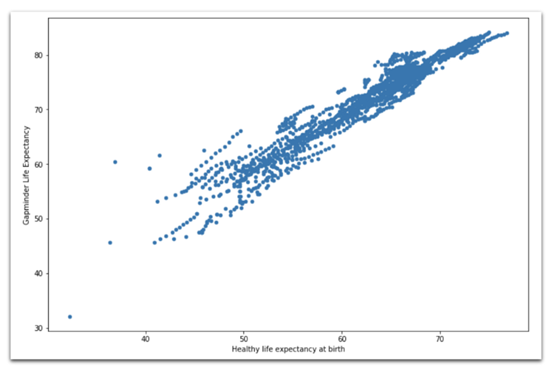Hexbin图

data[data['Year'] == 2018].plot(    kind='hexbin',    x='Healthy life expectancy at birth',    y='Generosity',    C='Life Ladder',    gridsize=20,    figsize=(12,8),    cmap="Blues", # defaults togreenish    sharex=False # required to get rid ofa bug)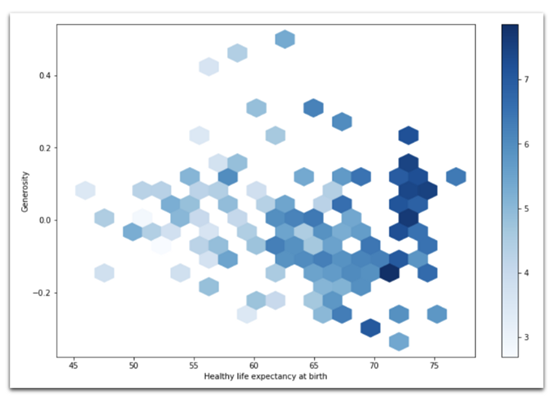2018年：Hexbin图，表示人的平均寿命与慷慨程度之间的关系。格子的颜色表示每个格子的平均寿命。

data[data['Year'] == 2018].groupby(    ['Continent'])['Gapminder Population'].sum().plot(    kind='pie',    figsize=(12,8),    cmap="Blues_r", # defaultsto orangish)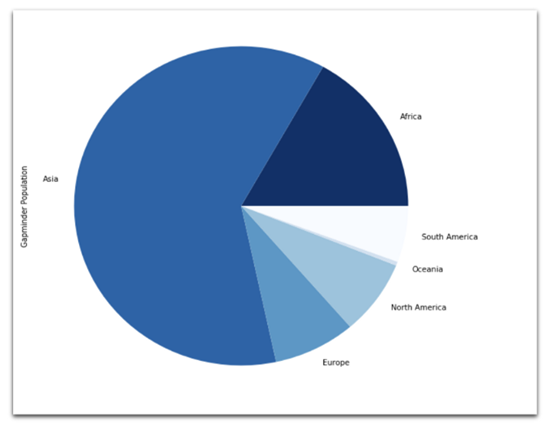2018年：按大洲划分的总人口数饼状图

data.groupby(    ['Year','Continent'])['Gapminder Population'].sum().unstack().plot(    kind='area',    figsize=(12,8),    cmap="Blues", # defaults toorangish)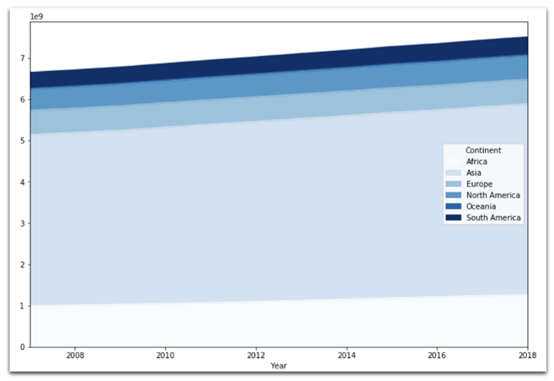data[    data['Country name'] == 'Germany'].set_index('Year')['Life Ladder'].plot(    kind='line',    figsize=(12,8))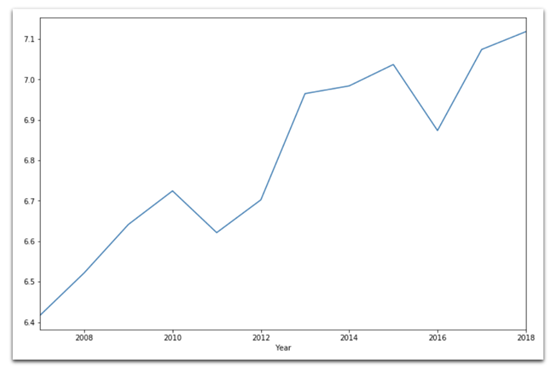# 5. 美观：使用Seaborn进行高级绘图Seaborn使用的是默认绘图。要确保运行结果与本文一致，请运行以下命令。

sns.reset_defaults()sns.set(    rc={'figure.figsize':(7,5)},    style="white" # nicerlayout)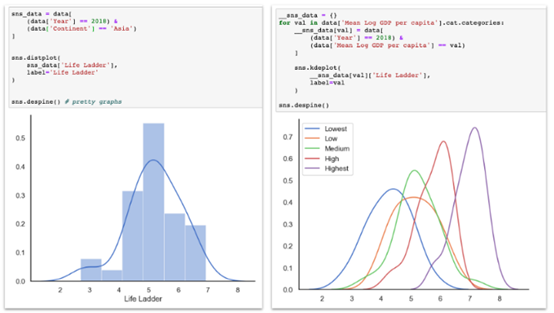sns.jointplot(    x='Log GDP per capita',    y='Life Ladder',    data=data,    kind='scatter' # or 'kde' or 'hex')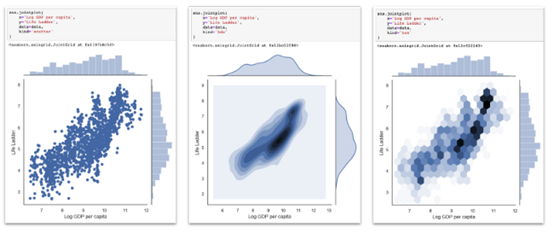Seaborn双标图，散点图、二元KDE和Hexbin图都在中心图中，边缘分布在中心图的左侧和顶部。

sns.scatterplot(    x='Log GDP per capita',    y='Life Ladder',    data=data[data['Year'] == 2018],       hue='Continent',    size='Gapminder Population')# both, hue and size are optionalsns.despine() # prettier layout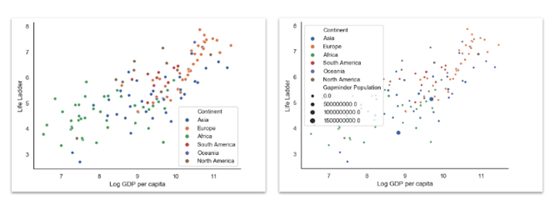sns.set(    rc={'figure.figsize':(18,6)},    style="white")sns.violinplot(    x='Continent',    y='Life Ladder',    hue='Mean Log GDP per capita',    data=data)sns.despine()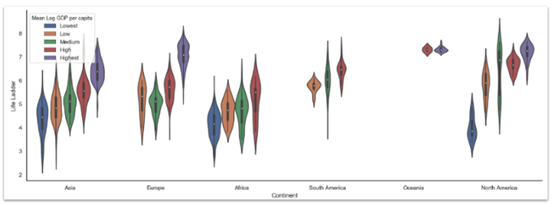Seaborn配对图是在一个大网格中绘制双变量散点图的所有组合。我通常觉得这有点信息过载，但它有助于发现规律。

sns.set(    style="white",    palette="muted",    color_codes=True)sns.pairplot(    data[data.Year == 2018][[        'Life Ladder','Log GDP percapita',        'Social support','Healthy lifeexpectancy at birth',        'Freedom to make lifechoices','Generosity',        'Perceptions of corruption','Positive affect',        'Negative affect','Confidence innational government',        'Mean Log GDP per capita'    ]].dropna(),    hue='Mean Log GDP per capita')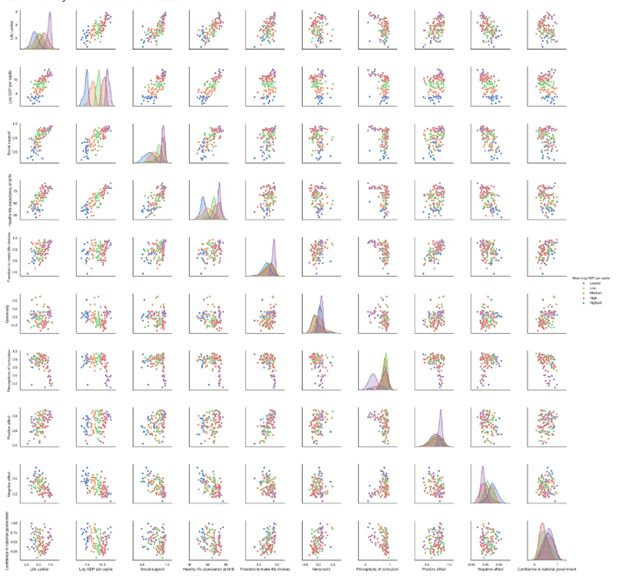Seaborn散点图网格中，所有选定的变量都分散在网格的下半部分和上半部分，对角线包含Kde图。

FacetGrids

FacetGrid— 折线图

g = sns.FacetGrid(    data.groupby(['Mean Log GDP percapita','Year','Continent'])['Life Ladder'].mean().reset_index(),    row='Mean Log GDP per capita',    col='Continent',    margin_titles=True)g = (g.map(plt.plot, 'Year','Life Ladder'))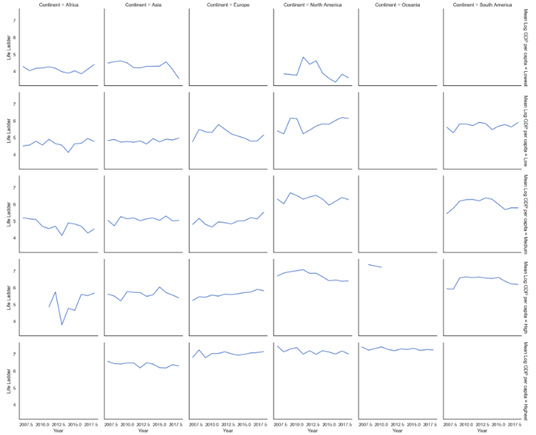y轴代表生活阶梯，x轴代表年份。网格的列代表大洲，网格的行代表不同水平的人均GDP。总体而言，北美人均GDP平均值较低的国家和欧洲人均GDP平均值中等或较高的国家，情况似乎有所好转

FacetGrid— 直方图

g = sns.FacetGrid(data,col="Continent", col_wrap=3,height=4)g = (g.map(plt.hist, "Life Ladder",bins=np.arange(2,9,0.5)))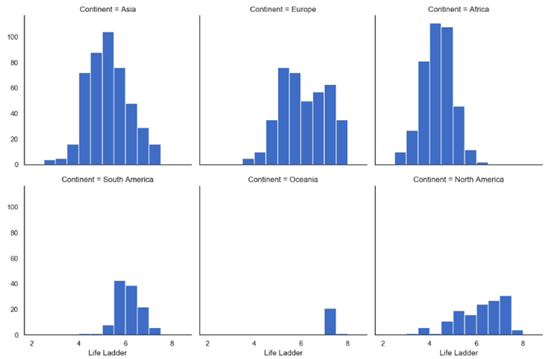FacetGrid— 带注释的KDE图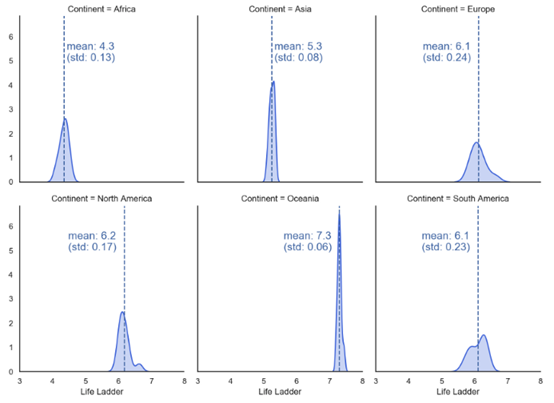defvertical_mean_line(x, **kwargs):    plt.axvline(x.mean(), linestyle="--",                color= kwargs.get("color", "r"))    txkw =dict(size=15, color= kwargs.get("color", "r"))    label_x_pos_adjustment =0.08# this needs  customization based on your data    label_y_pos_adjustment =5# this needs  customization based on your data    if x.mean() <6: # this needs customization based on  your data        tx ="mean: {:.2f}\n(std: {:.2f})".format(x.mean(),x.std())        plt.text(x.mean() +  label_x_pos_adjustment, label_y_pos_adjustment, tx, **txkw)    else:        tx ="mean: {:.2f}\n   (std: {:.2f})".format(x.mean(),x.std())        plt.text(x.mean() -1.4,  label_y_pos_adjustment, tx, **txkw)_ =  data.groupby(['Continent','Year'])['Life Ladder'].mean().reset_index()g =  sns.FacetGrid(_, col="Continent", height=4, aspect=0.9, col_wrap=3, margin_titles=True)g.map(sns.kdeplot,  "Life  Ladder", shade=True, color='royalblue')g.map(vertical_mean_line,  "Life  Ladder")
annotate_facet_grid.py hostedwith ❤ by GitHub

FacetGrid— 热图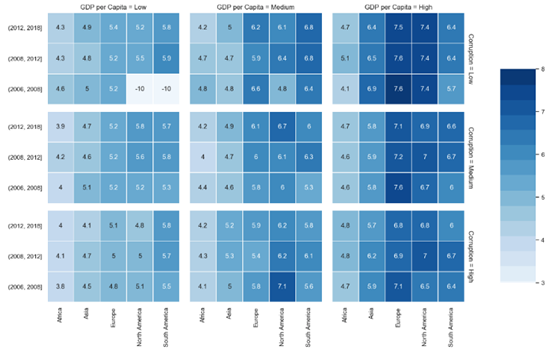Facet热图，外层的行显示在一年内，外层的列显示人均GDP，内层的行显示政治清廉，内层的列显示大洲。我们看到幸福指数朝着右上方向增加（即，高人均GDP和高政治清廉）。时间的影响还不确定，一些大洲（欧洲和北美）似乎比其他大洲（非洲）更幸福。

heatmap_facetgrid.py
defdraw_heatmap(data,inner_row,  inner_col, outer_row, outer_col, values, vmin,vmax):    sns.set(font_scale=1)    fg = sns.FacetGrid(        data,        row=outer_row,        col=outer_col,        margin_titles=True    )    position = left, bottom, width, height =1.4, .2, .1, .6    cbar_ax = fg.fig.add_axes(position)    fg.map_dataframe(        draw_heatmap_facet,        x_col=inner_col,        y_col=inner_row,        values=values,        cbar_ax=cbar_ax,        vmin=vmin,        vmax=vmax    )    fg.fig.subplots_adjust(right=1.3)     plt.show()defdraw_heatmap_facet(*args, **kwargs):    data = kwargs.pop('data')    x_col = kwargs.pop('x_col')    y_col = kwargs.pop('y_col')    values = kwargs.pop('values')    d = data.pivot(index=y_col, columns=x_col, values=values)    annot =round(d,4).values    cmap = sns.color_palette("Blues",30) +  sns.color_palette("Blues",30)[0::2]    #cmap =  sns.color_palette("Blues",30)    sns.heatmap(        d,        **kwargs,        annot=annot,        center=0,        cmap=cmap,        linewidth=.5    )# Data  preparation_ = data.copy()_['Year'] = pd.cut(_['Year'],bins=[2006,2008,2012,2018])_['GDP per  Capita'] = _.groupby(['Continent','Year'])['Log GDP per  capita'].transform(    pd.qcut,    q=3,    labels=(['Low','Medium','High'])).fillna('Low')_['Corruption'] = _.groupby(['Continent','GDP per  Capita'])['Perceptions  of corruption'].transform(    pd.qcut,    q=3,    labels=(['Low','Medium','High']))_ = _[_['Continent'] !='Oceania'].groupby(['Year','Continent','GDP per  Capita','Corruption'])['Life Ladder'].mean().reset_index()_['Life Ladder'] = _['Life Ladder'].fillna(-10)draw_heatmap(    data=_,    outer_row='Corruption',    outer_col='GDP per Capita',    inner_row='Year',    inner_col='Continent',    values='Life Ladder',    vmin=3,    vmax=8,)
heatmap_facetgrid.py hostedwith ❤ by GitHub# 6. 精彩：用plotly创造精彩的互动情节·     悬停：当鼠标悬停在图表上时，会弹出注释
·     交互性：不需要任何额外设置，图表就可以进行交互（例如，一次穿越时间的旅程）
·     漂亮的地理空间图：Plotly已经内置了一些基本的映射功能，另外，还可以使用mapbox集成来制作令人惊叹的图表。

 fig = x.<PLOTTYPE>(PARAMS)然后是 fig.show() ，像这样：fig = px.scatter(    data_frame=data[data['Year'] ==2018],    x="Log GDP per capita",    y="Life Ladder",    size="GapminderPopulation",    color="Continent",    hover_name="Country name",    size_max=60)fig.show()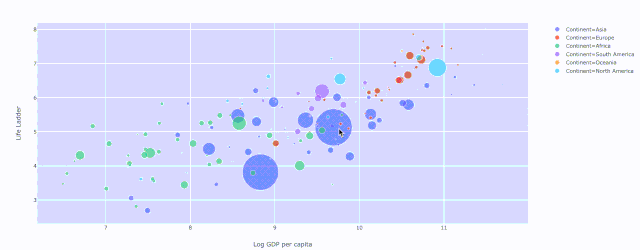Plotly散点图，绘制人均 GDP与生活阶梯的关系，其中颜色表示大洲和人口的大小

fig = px.scatter(    data=data,    x="Log GDP per capita",    y="Life Ladder",    animation_frame="Year",    animation_group="Countryname",    size="GapminderPopulation",    color="Continent",    hover_name="Country name",    facet_col="Continent",    size_max=45,   category_orders={'Year':list(range(2007,2019))}    )fig.show()def q_bin_in_3(col):    return pd.qcut(        col,        q=3,        labels=['Low','Medium','High']    )_ = data.copy()_['Social support'] = _.groupby('Year')['Socialsupport'].transform(q_bin_in_3)_['Life Expectancy'] =_.groupby('Year')['Healthy life expectancy atbirth'].transform(q_bin_in_3)_['Generosity'] =_.groupby('Year')['Generosity'].transform(q_bin_in_3)_['Perceptions ofcorruption'] = _.groupby('Year')['Perceptions ofcorruption'].transform(q_bin_in_3)_ = _.groupby(['Social support','LifeExpectancy','Generosity','Perceptions of corruption'])['LifeLadder'].mean().reset_index()fig = px.parallel_categories(_, color="LifeLadder", color_continuous_scale=px.colors.sequential.Inferno)fig.show()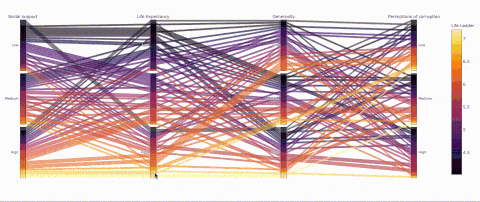fig = px.bar(    data,    x="Continent",    y="Gapminder Population",    color="Mean Log GDP percapita",    barmode="stack",    facet_col="Year",    category_orders={"Year":range(2007,2019)},    hover_name='Country name',    hover_data=[        "Mean Log GDP percapita",        "Gapminder Population",        "Life Ladder"    ])fig.show()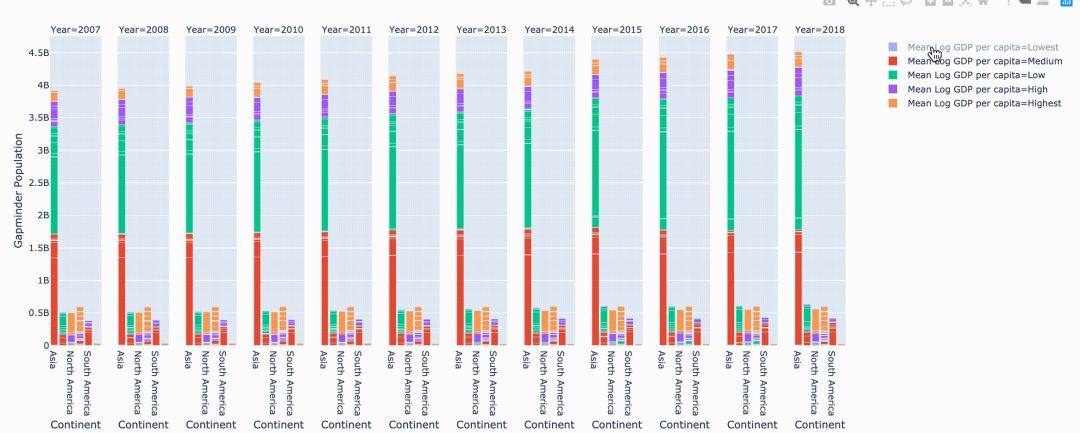fig = px.choropleth(    data,    locations="ISO3",    color="Life Ladder",    hover_name="Country name",   animation_frame="Year")fig.show()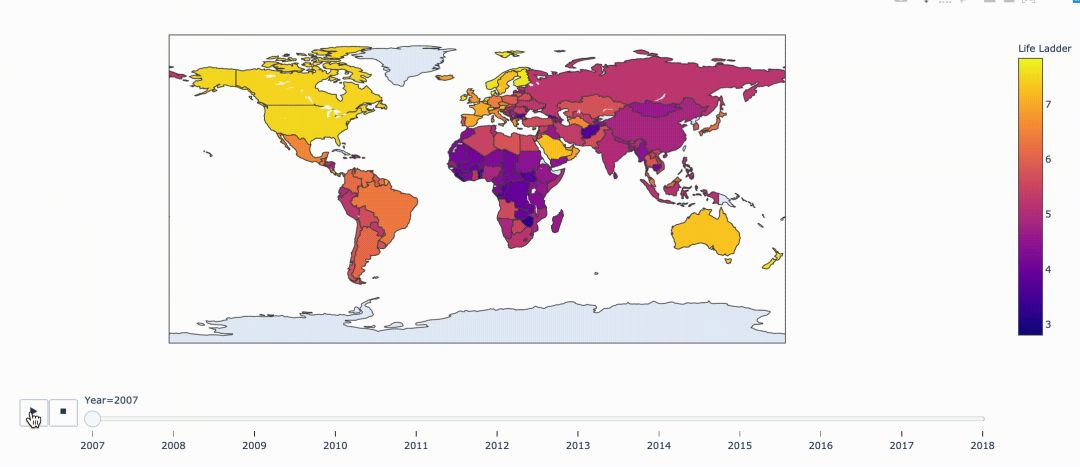# 结束语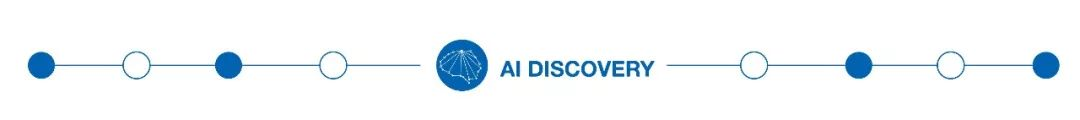https://towardsdatascience.com/plotting-with-python-c2561b8c0f1f

ACL2018论文集50篇解读
EMNLP2017论文集28篇论文解读
2018年AI三大顶会中国学术成果全链接
ACL2017论文集：34篇解读干货全在这里
10篇AAAI2017经典论文回顾©️2019 CSDN 皮肤主题: 大白 设计师: CSDN官方博客